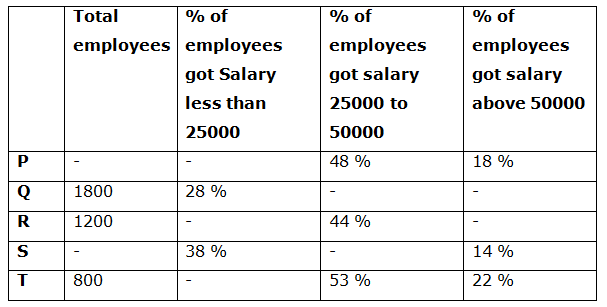# NIACL AO Mains– Quantitative Aptitude Questions Day- 20

Dear Readers, Bank Exam Race for the Year 2019 is already started, To enrich your preparation here we have providing new series of Practice Questions on Quantitative Aptitude – Section. Candidates those who are preparing for NIACL AO Mains 2019 Exams can practice these questions daily and make your preparation effective.

[WpProQuiz 5280]

Directions (1 – 5): What value should come in the place of question mark (?) in the following questions?

1) 586 × 9234 ÷ 48 % of 950 = 36 × 750 ÷ 4 + ? + 4500

a) 546.25

b) 728

c) 616.5

d) 832.75

e) 474

2) 54 % of 650 +? % of 120 – 40 % of 8880 = 7293

a) 6890

b) 7865

c) 9270

d) 8745

e) 5620

3) (3/7) of 245 × 135 ÷ 15 – ? = 528 ÷ 3 + 217

a) 624

b) 552

c) 786

d) 678

e) 580

4) 12 (¾) % of 2400 + 23 (½) % of 800 + 452 = 1125 ÷ 25 + 4 × ?

a) 658.25

b) 532.5

c) 618.5

d) 614.75

e) 590.5

5) (12/75) of (48/1136) of (32/96) of 10650 = ? % of 500

a) 4.8

b) 6.2

c) 7.4

d) 8.6

e) None of these

Directions (6 – 10): Study the following graph carefully and answer the given question.

The following table shows the total number of employees in different companies and the percentage of employees who got their salary less than 25000, 25000 to 50000 and above 50000 respectively. Some values are missing here.Note:

Total employees = % of people got Salary less than 25000 + % of people got salary 25000 to 50000 + % of people got salary above 50000

6) Find the difference between the total employees who got salary less than 25000 from company P to that of company R, if the ratio of total employees in company P to that of Q is 5 : 6 and the percentage of employees who got salary above 50000 from company R is 24 %?

a) 258

b) 272

c) 126

d) 184

e) None of these

7) Find the sum of the total number of employees who got salary 25000 to 50000 from company Q, R and T together, if the percentage of employees who got salary above 50000 from company Q is 15 %?

a) 1978

b) 1526

c) 1642

d) 1890

e) None of these

8) Find the average number of employees who got their salary less than 25000 in company P, Q and S together, if the total number of employees who got their salary above 50000 from company P is 270 and the total number of employees in company S is twice the number of employees in company R?

a) 578

b) 776

c) 694

d) 642

e) None of these

9) Total employees in company Q and R together is what percentage of total employees in company S and T together, if the total number of employees who got their salary 25000 to 50000 from company S is 1152?

a) 82.5 %

b) 104.25 %

c) 93.75 %

d) 116.25 %

e) None of these

10) Find the ratio between the total number of employees who got salary less than 25000 from company T to that of total number of employees who got salary above 25000 from company Q, if the number of employees who got salary 25000 from company Q is 96?

a) 2: 7

b) 1: 6

c) 3: 8

d) 4: 11

e) None of these

Direction (1-5) :

586 × [9234 ÷ ((48/100)*950)] = 36 × (750/4) + x + 4500

586*(9234/456) = 6750 + x + 4500

11866.5 – 11250

X = 616.5

(54/100)*650 + (x/100)*120 – (40/100)*8880 = 7293

351 + 6x/5 – 3552 = 7293

6x/5 = 7293 – 351 + 3552

6x/5 = 10494

X = 10494*(5/6) = 8745

(3/7)*245*(135/15) + x = (528/3) + 217

945 – x = 176 + 217

X = 945 – 176 – 217

X = 552

(51/400)*2400 + (47/200)*800 + 2025 = (1125/25) + 4x

306 + 188 + 2025 – 45 = 4x

2474 = 4x

X = 2474/4 = 618.5

(12/75)* (48/1136)*(32/96) * 10650 = (x/100)*500

24 = 5x

X = 24/5 = 4.8

Direction (6-10) :

The total employees who got salary less than 25000 from company P

= > (1800/6)*5*(34/100) = 510

The total employees who got salary less than 25000 from company R

= > 1200*(32/100) = 384

Required difference = 510 – 384 = 126

The total number of employees who got salary 25000 to 50000 from company Q, R and T together

= > 1800*(57/100) + 1200*(44/100) + 800*(53/100)

= > 1026 + 528 + 424 = 1978

The total number of employees who got their salary above 50000 from company P = 270

(18/100)*total employees in company P = 270

Total employees in company P = 270*(100/18) = 1500

The total number of employees who got their salary less than 25000 in company P, Q and S together

= > 1500*(34/100) + 1800*(28/100) + (2*1200)*(38/100)

= > 510 + 504 + 912 = 1926

Required average = 1926/3 = 642

The total number of employees who got their salary 25000 to 50000 from company S = 1152

Total employees in company S = 1152*(100/48) = 2400

Total employees in company Q and R together

= > 1800 + 1200 = 3000

The total employees in company S and T together

= > 2400 + 800 = 3200

Required % = (3000/3200)*100 = 93.75 %

The total number of employees who got salary less than 25000 from company T

= > 800*(25/100) = 200

The total number of employees who got salary above 25000 from company Q

= > 1800*(72/100) – 96 = 1296 – 96 = 1200

Required ratio = 200: 1200 = 1: 6